# PSAT Math : How to find the equation of a perpendicular line

## Example Questions

1 3 Next →

### Example Question #1 : How To Find The Equation Of A Perpendicular Line

If a line has an equation of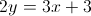, what is the slope of a line that is perpendicular to the line?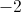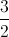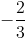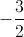Explanation:

Putting the first equation in slope-intercept form yields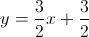.

A perpendicular line has a slope that is the negative inverse. In this case,.

### Example Question #12 : How To Find The Equation Of A Perpendicular Line

Which of the following equations represents a line that goes through the pointand is perpendicular to the line?Explanation:

In order to solve this problem, we need first to transform the equation from standard form to slope-intercept form:Transform the original equation to find its slope.First, subtractfrom both sides of the equation.Simplify and rearrange.Next, divide both sides of the equation by 6.The slope of our first line is equal to. Perpendicular lines have slopes that are opposite reciprocals of each other; therefore, if the slope of one is x, then the slope of the other is equal to the following:Let's calculate the opposite reciprocal of our slope:The slope of our line is equal to 2. We now have the following partial equation:We are missing the y-intercept,. Substitute the x- and y-values in the given pointto solve for the missing y-intercept.Add 4 to both sides of the equation.Substitute this value into our partial equation to construct the equation of our line:1 3 Next →